# How to do case sensitive lookup

A case-sensitive lookup can be done using an array of functions like INDEX, MATCH, and EXACT. Always remember that you need to enter the formula with the array of functions using the Control + Shift +Enter. Note that the EXACT function will always return TRUE if there are two similar strings. The same function turns FALSE if otherwise.

There are two other methods to do the case-sensitive lookup and they are using the VLOOKUP, CHOOSE formula, and using the LOOKUP. This article will look at each formula and how it can be used to do a case-sensitive lookup. VLOOKUP does not differentiate between CAPS and small letters making it bad for case-sensitive lookup. That shows that VLOOKUP in excel is used for case insensitive lookup because It does not tell the difference between small and capital letters.

Let us look at a step-by-step guide on how to do a case-sensitive lookup using EXACT, MATCH, and INDEX.

How to use EXACT, INDEX, MATCH to do a case sensitive lookup

Assuming we have the salaries of 7 people but two people share the same name. In our excel sheet, we save one employee with small caps (peter) and the second employee as (PETER). We can use EXACT, MATCH, and INDEX to form an array that will look up the salary of PETER. Open a blank excel document and type in a list of seven people. One name should appear twice, as in small letters and CAPS. Create another row and add the salaries. Now follow these simple steps to do a case-sensitive lookup of the repeated name.

1. =Exact(E3,A2) returns FALSE because the string PETER and peter are not exactly the same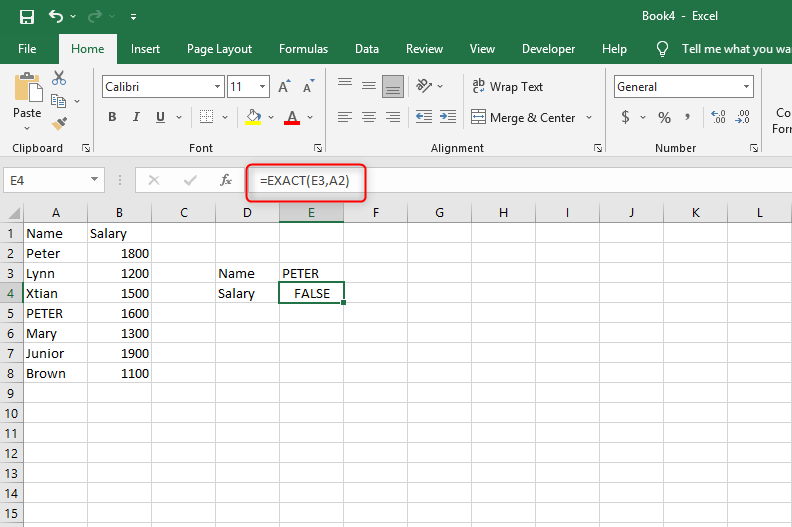2. =Exact(E3,A5) returns TRUE because the string PETER and PETER strings are exactly the same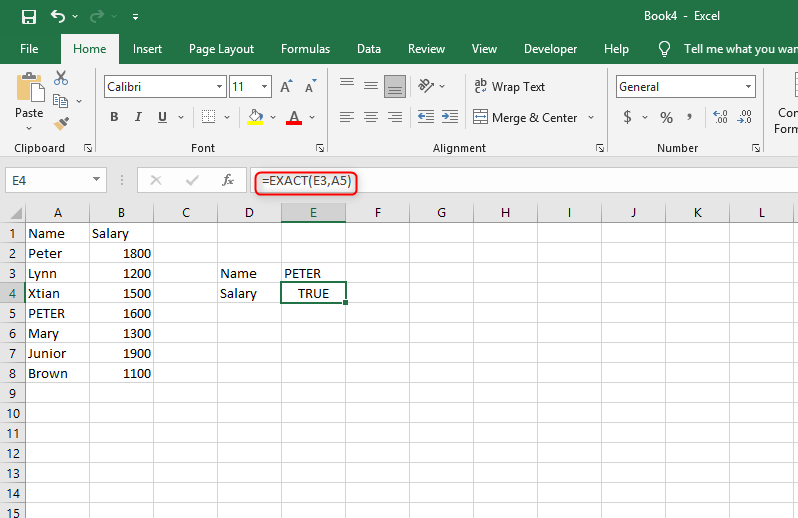3. Now let us replace A5 with A2:A8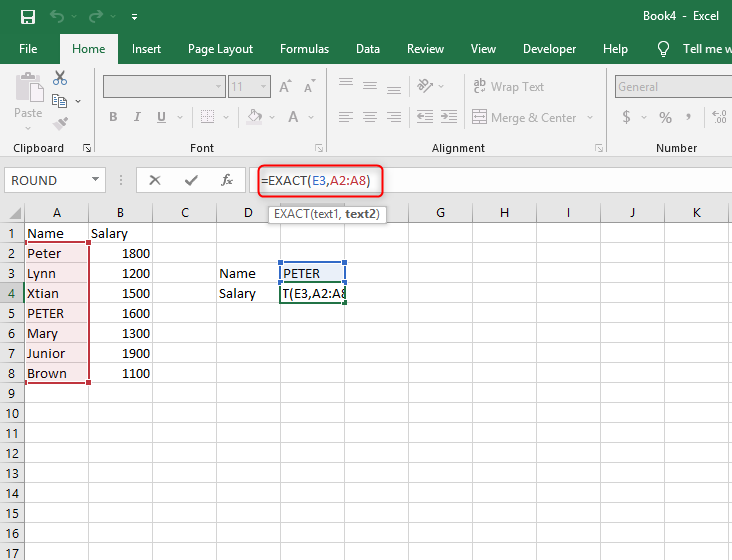Now our array can look for matching strings through the whole row. The array constant will be as follows

{FALSE;FALSE;FALSE;TRUE;FALSE;FALSE;FALSE}

4. .In this step we will add a function that will find the position of TRUE from the array. Note that MATCH will always return the first value. For instance, we have another PETER in our lists, there will be two TRUE in the array Match will return the first matching string.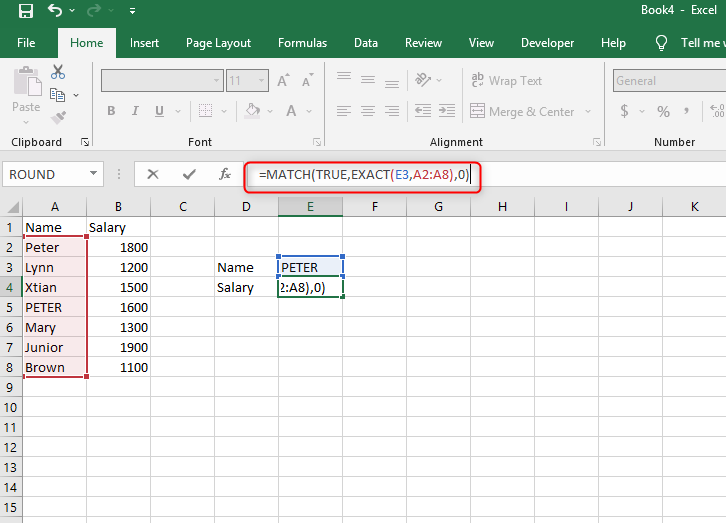5. Press ctrl+shift+enter since it's an array formula.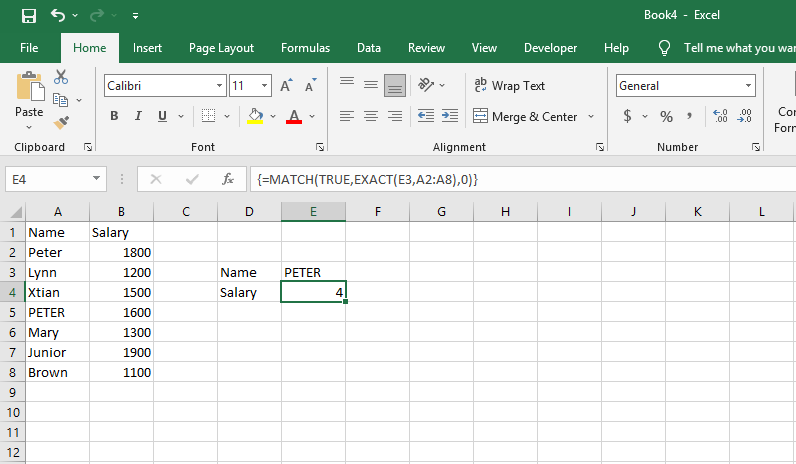4 is the TRUE position in the array

6. Let's use the INDEX function to return a specific value peter from the salary row. Index checks the corresponding salary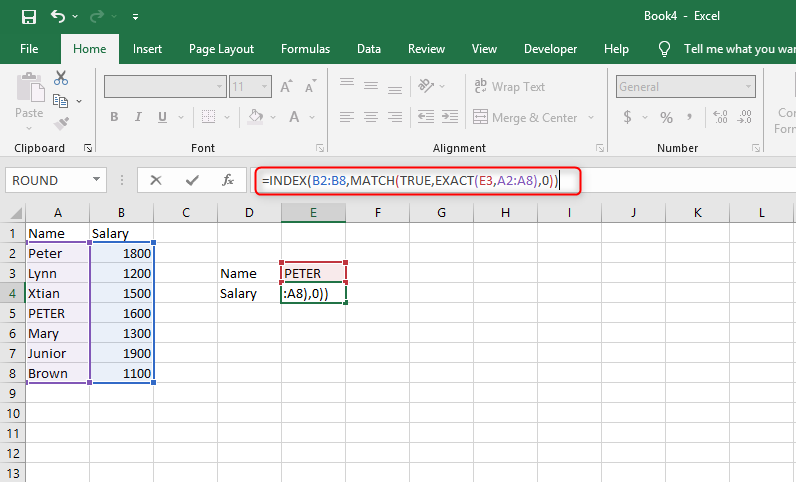7. Finish by pressing ctrl+shift+enter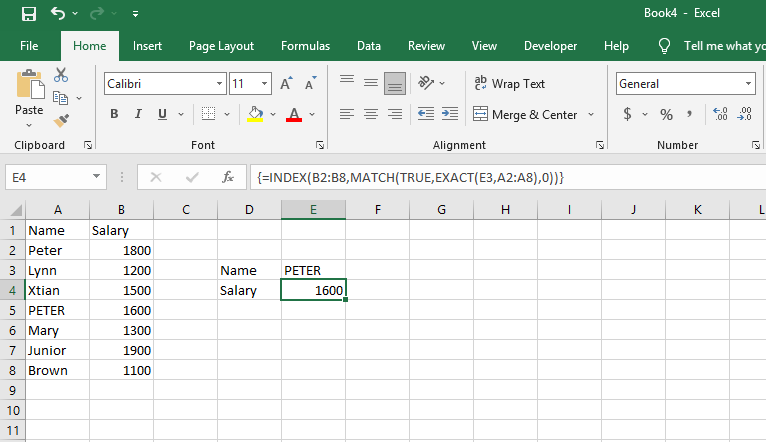On the formula bar, you will notice that the formula is enclosed with braces indicating that, you have used an array of formulas. Do not type the formula yourself, just type in the keys and replace the necessary and you will have done your case-sensitive lookup successfully.

Final thoughts

VLookup is not suitable to do a case-sensitive lookup. However, we can use, Index, match, and exact to form an array that looks up the exact match of a string. This is useful when you want to look up an exact code, salary, employees, and so on from a long list. The only downside of using this formula is that it returns only the first match.